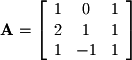Matrices are another fundamental concept to linear algebra. A matrix is a rectangular table of entries. The notation for a matrix is generally a bold-faced capital letter such as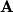. Matrices in introductory linear algebra generally have 2 dimensions. The notation for the size of a matrix is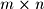(pronounced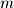by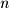) whereis the number of horizontal rows andis the number of vertical columns. For example, the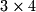matrix shown in Figure 1-9 has 3 horizontal rows and 4 vertical columns.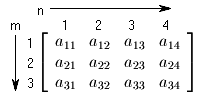Figure 1-9.
Matrix withrows andcolumns shown
The row by column notation is easy to mix up (i.e. to write it backwards as column by row). If you have trouble remembering it, think of it this way... we'll call it the "page method." Say that you want to identify the fourth word in third line of the top paragraph in this page with a row-column index. The most intuitive way would be to count down 3 lines (rows) and over by 4 words (columns). In this situation, doing it backwards (counting over 4 words first and then 3 lines down) does not make sense because there are different numbers of words on each line. Although matrices always have the same number of columns on every row, you can use the "page method" to remember that the order is row number first and column number second.

You can also see in Figure 1-9 that the entries are specified by a lower case letter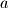with a subscript index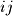. For example for a matrix, the entry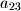(pronounced "a-two-three") is the value in the 2nd row and 3rd column.

A common way to write a matrix of arbitrarydimensions in formal notation is shown below: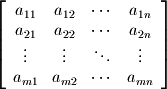Excercise 1-1.
Determine the following entries given the matrix: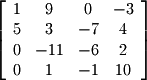1.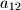2.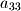3.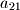4.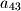An ordered collection of vectors (unlike a set which is an unordered collection of vectors) can be grouped into a matrix which will be useful for later. For example, the three vectors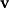,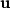, and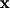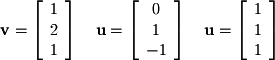can be grouped as the following matrix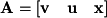: